# Consider vertical upward and horizontal to right-hand side to be positive orientations. The below truss is...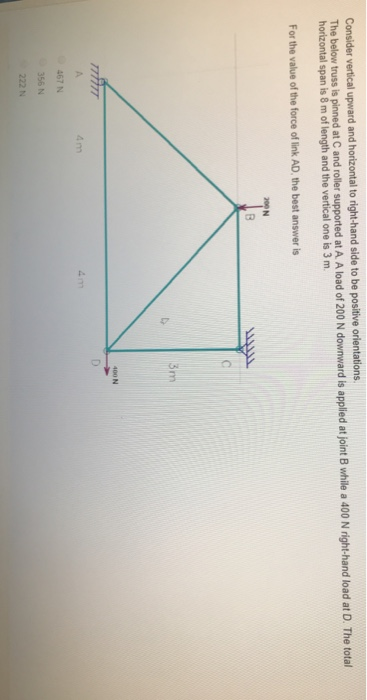Consider vertical upward and horizontal to right-hand side to be positive orientations. The below truss is pinned at C and roller supported at A. A load of 200 N downward is applied at joint B while a 400 N right-hand load at D. The total horizontal span is 8 m of length and the vertical one is 3 m. For the value of the force of link AD, the best answ er is 200 N 3m 400 N D A 4m 4m 467 N 356 N 222 N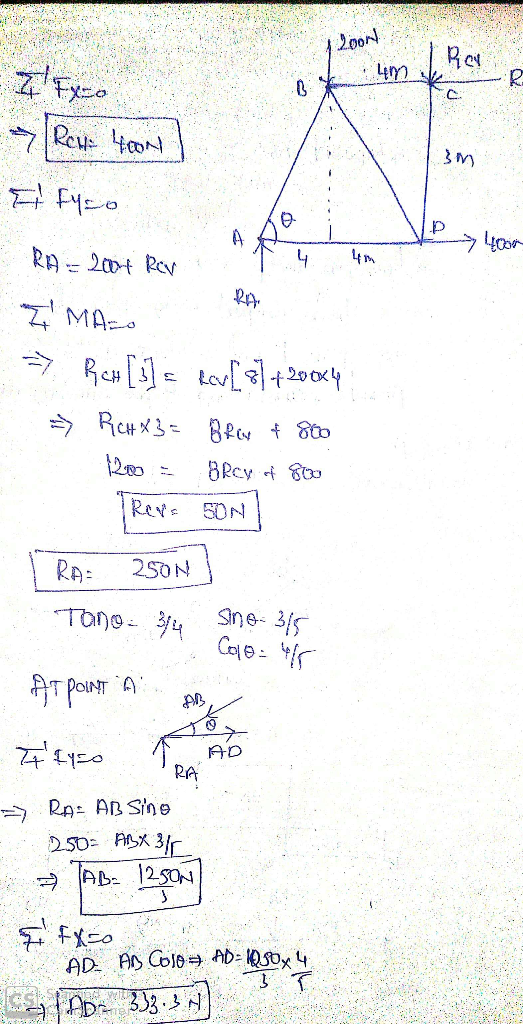#### Earn Coin

Coins can be redeemed for fabulous gifts.

Similar Homework Help Questions
• ### Tarnororaovore oorRo oroor wrram The below truss is pinned at C and roller supported at A....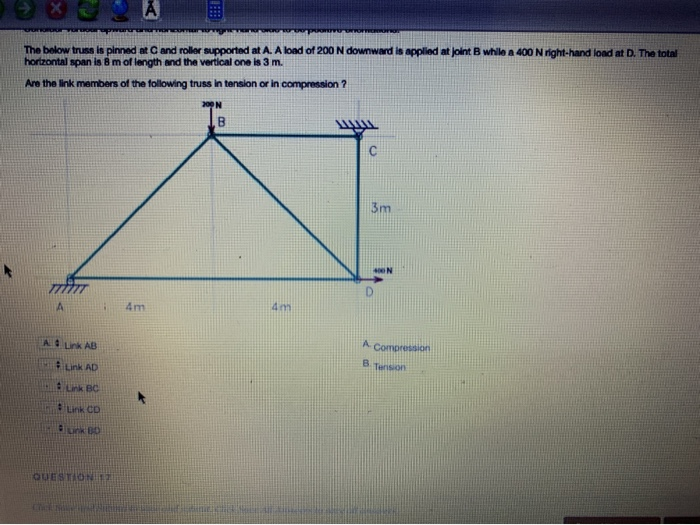Tarnororaovore oorRo oroor wrram The below truss is pinned at C and roller supported at A. A load of 200 N downward is applied at joint B while a 400 N right-hand load at D. The total horizontal span is 8 m of length and the vertical one is 3 m. or in compression ? Are the link members of the following truss in tension 00N 3m 400N 4m 4m A Compression Ad Lnk AB B Tension Link AD nk...

• ### 2. Determine the vertical displacement at joint B and horizontal displacement at joint D using Castigliano's...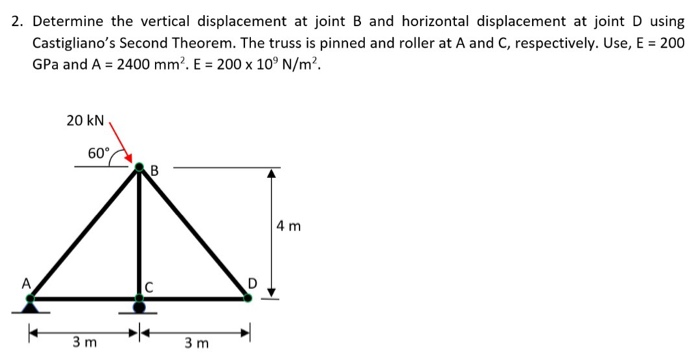2. Determine the vertical displacement at joint B and horizontal displacement at joint D using Castigliano's Second Theorem. The truss is pinned and roller at A and C, respectively. Use, E = 200 GPa and A = 2400 mm. E = 200 x 10°N/m². 20 KN 60 CAB 4 m 3m 3m

• ### A 3 m rigid bar AB is supported with a vertical translational spring at A and a pin at B The bar is subjected to a linearly varying distributed load with maximum intensity g Calculate the ver...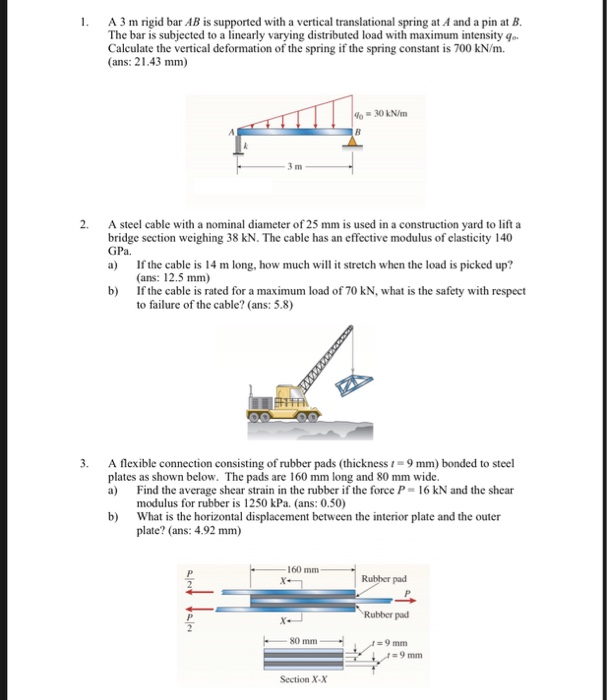A 3 m rigid bar AB is supported with a vertical translational spring at A and a pin at B The bar is subjected to a linearly varying distributed load with maximum intensity g Calculate the vertical deformation of the spring if the spring constant is 700 kN/m. (ans: 21.43 mm) 2. A steel cable with a nominal diameter of 25 mm is used in a construction yard to lift a bridge section weighing 38 kN. The cable has an...

Free Homework App